# Brain Teaser :: Can You Solve the iF Then Puzzle?

in STEMGeeks3 months ago

Hey All; @StemGeeks Mathematician;

Without further, ado, let's get to our Brain Teaser :: Can You Solve the iF Then Puzzle?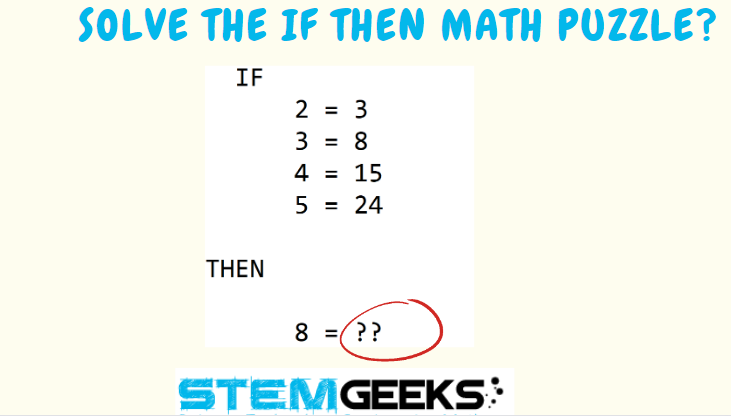Have a close look at the image given and see if you can find the missing number in the last line. Just remember there hasn't have to be a logic always. The puzzle isn't that hard to solve.

There are few different solution and simple basic math's knowledge is required to get the equation right. Just give it a try - all you need to do is pick up a rough paper and see the equation that's being formed and you will get the answer. Just give it a try and its that simple I assure you. There is no right or wrong - comment your answer on the reply box below to enter a chance to WIN 10 STEM tokens...

### STEM token GiveAway...

I'll be again doing a giveaway of STEM tokens to the lucky random winner with the correct answer. For the last contest, which was Brain Teaser :: Solve the math puzzle?.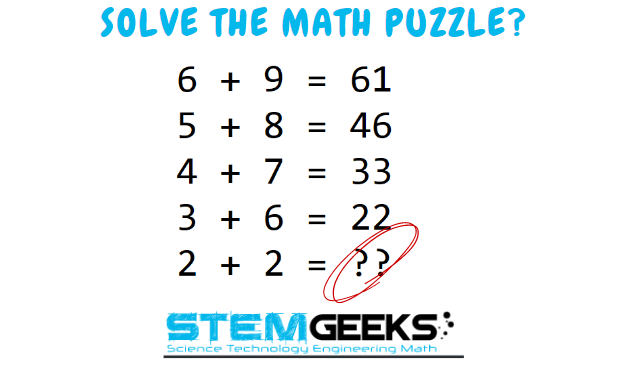### Solution :: Brain Teaser :: Solve the math puzzle?

###### Pattern being followed was:: X * Y + (X + 1) = Answer = That Simple it was - i.e.::
• First Row :: 6 x 9 + (6 + 1) = 61

• Second Row :: 5 x 8 + (5 + 1) = 46

• Third Row :: 4 x 7 + (4 + 1) = 33

• Fourth Row :: 3 x 6 + (3 + 1) = 22

• Fifth Row :: 2 x 2 + (2 + 1) = 7

Finally the answer to the puzzle was:: 7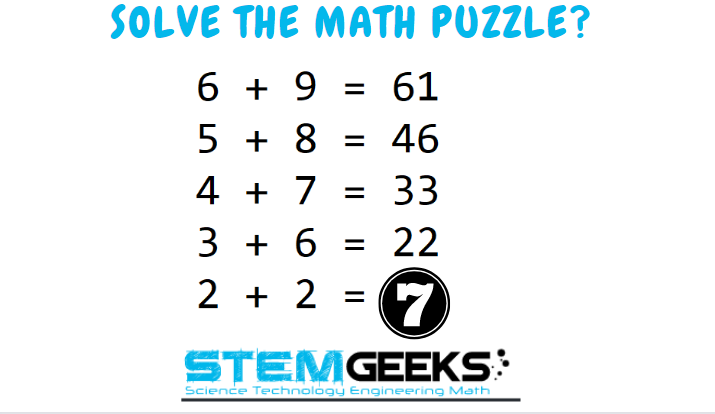We had three entries and all of them provided the right answer. Hence, I am going to Split the rewards amongst all the three winners - @beulah4real, @lovefallen and @lochard... And the Winner is ::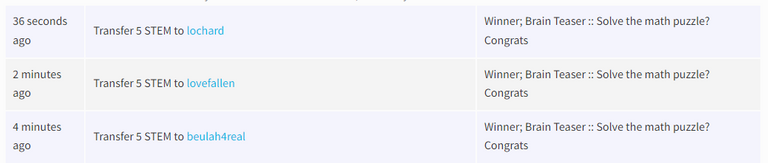Congratulations ALL - You all WIN yourself 5 STEM tokens each. You should be having the rewards in your STEM Wallet Soon.

### Math Quote for the Day::

Here is the motivation to solve the Math Puzzle?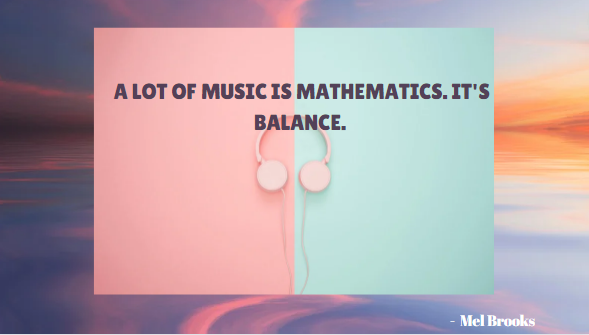If you like my work, then please spread the Word.. that we do have the Math Brain Teasers competition here @StemGeeks platform. Reblog is much appreciated.

Best Regards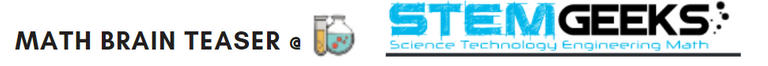Find Me on the Other Social Media Platforms::PS:: All the Maths Brain Teasers; are made by me using the Pro Canva License Version

Sort:

I bet on 63.
But of course it can also be 3? Because 3 = 8

Btw, what are STEM tokens? Do I need to create a STEM wallet?